## Test of exotic scalar and tensor couplings in K+ → π0e+ν decay

### Motivation

After the discovery of parity violation in nuclear decay of polarized 60Co in 1957, many experimental and theoretical works have been performed to understand the properties of the weak interaction. So far, the interaction based on the pure V-A coupling feature have been successful in explaining experimental observations in nuclear, meson , and muon decays. However, in 1991, scalar(S) and tensor(T) interactions were observed in K+ → π0e+ν (Ke3) decay. The existence of these exotic interactions is in distinct disagreement with the Standard Model and thus requires an accurate experimental check. In order to check this important result, we tested the S and T couplings in the Ke3 decay using the experimental apparatus which was constructed for a T-violation search in K+ → π0μ+ν decay.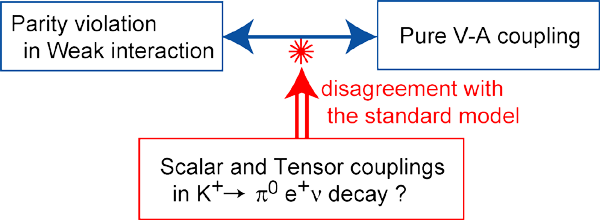### Event selection

Ke3 events were identified by analyzing the e+ momentum with the spectrometer and detecting two photons in the CsI(Tl) calorimeter. Charged particles from the target were tracked and momentum-analyzed using MWPCs at the entrance and exit of the magnet gap, as well as by the active target and an array of ring counters surrounding the target. The e+s were selected by measuring time-of-flight between TOF counters. The photon energy and hit position were obtained by summing the energy deposits and energy-weighted centroid, respectively. The solid line in Fig.2(a,c) shows the e+ energy spectrum while (b,d) shows the opening angle distribution of the accepted Ke3 events.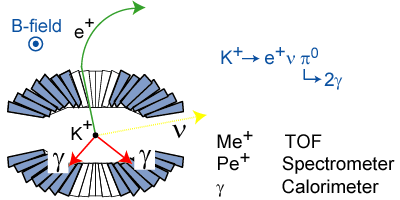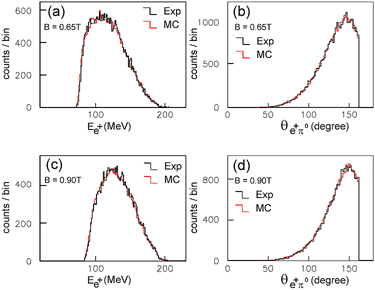Figure:1 Figure:2 Experimental spectra(black line) of Ee+(a, c) and θe+π0(b, d) for Ke3 decay, and Monte Carlo simulation (red line).

### Analysis

The most general Lorentz invariant form of the matrix element for the semi-leptonic K+ → π0l+ν (Kl3) decay is given by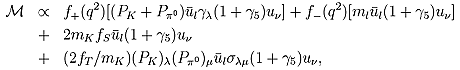where PK and Pπ0 are the four-momenta of the K+ and π0, respectively. f±(q2) are the usual vector form factors, which are functions of the momentum transfer squared q2 = (PK-Pπ0)2 to the leptons. fS and fT are the strengths of the potential scalar and tensor couplings, respectively. The vector form factor, assumed to depend linearly on q2 as f+(q2) = f+(0)[1+λ+ (q/mπ)2], and exotic form factors fS and fT could be extracted from the Dalitz plot distributions; parameters (λ+, fS/f+(0), fT/f+(0)) were determined by minimizing the difference between the observed Dalitz plot and a Monte Carlo simulation.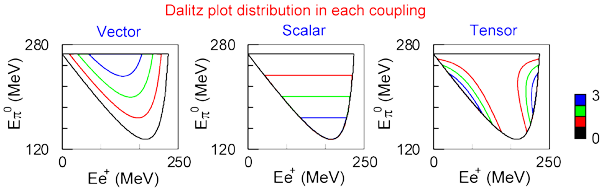### Results

The parameters are obtained to be ,

 λ+ = 0.0278±0.0026(stat)±0.0030(syst) fS/f+(0) = -0.002±0.026(stat)±0.014(syst) fT/f+(0) = -0.01±0.14(stat)±0.09(syst) .

The present value of the λ+ parameter is consistent with the current world average within experimental errors. fS/f+(0) and fT/f+(0) are consistent with the pure V-A coupling, which is not the case for the previous experiment.### Reference

 S.A. Akimenko et al., Phys. Lett. 259B, 225 (1991).
 M. Abe et al., Phys. Rev. Lett. 83, 4253 (1999).
 Particle Data Group, Eur. Phys. J. C15, Review of Particle Physics (2000).
 S. Shimizu et al. Phys. Lett. B495 (2000) 33.